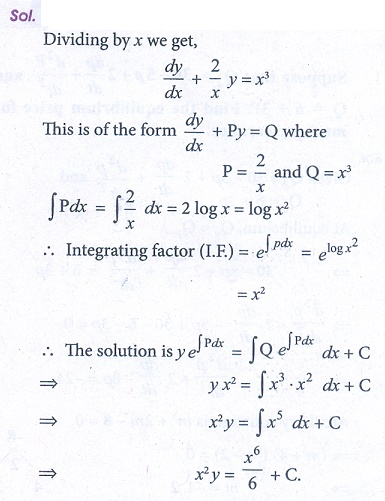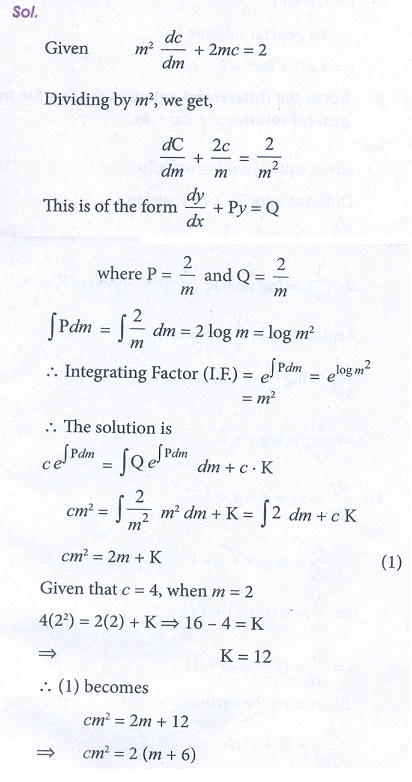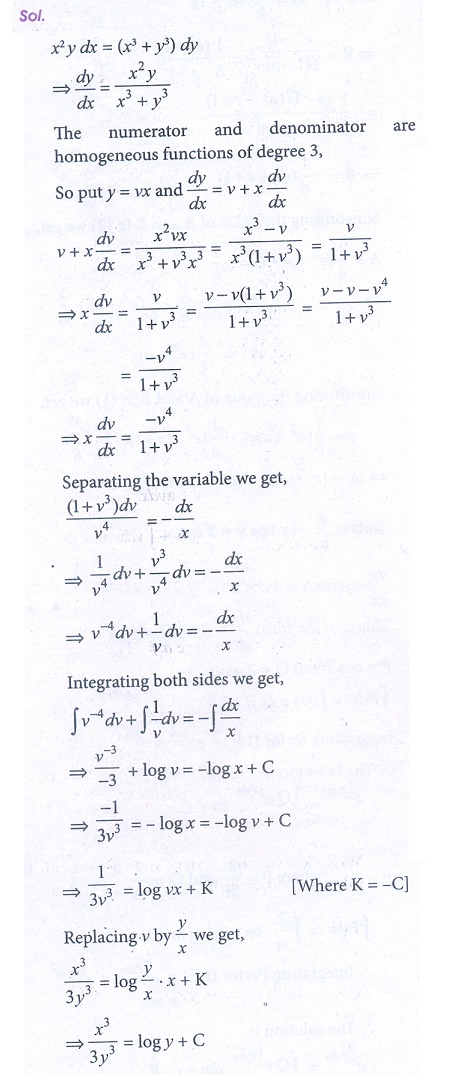Home | | Business Maths 12th Std | Miscellaneous Problems

# Miscellaneous Problems

Book back answers and solution for Exercise questions - Maths: Differential Equations: Miscellaneous Problems

Miscellaneous Problems

1. Suppose that Q = 30 − 5P + 2 dP/dt + d2P/dt2 and Qs = 6 + 3P . Find the equilibrium price for market clearance.2. Form the differential equation having for its general solution y = ax2 + bx3. Solve yx2dx + e−xdy = 04. Solve ( x2 + y2 )dx + 2xy dy = 05. Solve x dy/dx + 2 y = x46. A manufacturing company has found that the cost C of operating and maintaining the equipment is related to the length ‘m’ of intervals between overhauls by the equation m2 dC/dm + 2mC = 2 and c = 4 and when m = 2. Find the relationship between C and m.7. Solve ( D2 − 3D + 2)y = e4x given y = 0 when x = 0 and x = 1.8. Solve dy/dx + y cos x + x = 2 cos x9. Solve x2 ydx − ( x3 + y3 )dy = 010. Solve dy/dx = xy + x + y + 1